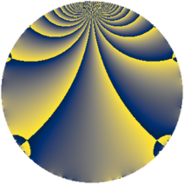# Properties

 Label 2169.2.aLevel $2169$ Weight $2$ Character orbit 2169.a Rep. character $\chi_{2169}(1,\cdot)$ Character field $\Q$ Dimension $100$ Newform subspaces $11$ Sturm bound $484$ Trace bound $2$

# Related objects

## Defining parameters

 Level: $$N$$ $$=$$ $$2169 = 3^{2} \cdot 241$$ Weight: $$k$$ $$=$$ $$2$$ Character orbit: $$[\chi]$$ $$=$$ 2169.a (trivial) Character field: $$\Q$$ Newform subspaces: $$11$$ Sturm bound: $$484$$ Trace bound: $$2$$ Distinguishing $$T_p$$: $$2$$

## Dimensions

The following table gives the dimensions of various subspaces of $$M_{2}(\Gamma_0(2169))$$.

Total New Old
Modular forms 246 100 146
Cusp forms 239 100 139
Eisenstein series 7 0 7

The following table gives the dimensions of the cuspidal new subspaces with specified eigenvalues for the Atkin-Lehner operators and the Fricke involution.

$$3$$$$241$$FrickeDim.
$$+$$$$+$$$$+$$$$14$$
$$+$$$$-$$$$-$$$$26$$
$$-$$$$+$$$$-$$$$33$$
$$-$$$$-$$$$+$$$$27$$
Plus space$$+$$$$41$$
Minus space$$-$$$$59$$

## Trace form

 $$100q + 2q^{2} + 102q^{4} + 4q^{7} + 6q^{8} + O(q^{10})$$ $$100q + 2q^{2} + 102q^{4} + 4q^{7} + 6q^{8} - 2q^{10} + 16q^{14} + 106q^{16} + 4q^{17} - 6q^{22} + 2q^{23} + 96q^{25} - 14q^{26} + 14q^{28} + 12q^{29} + 22q^{31} + 34q^{32} - 2q^{34} + 10q^{35} + 6q^{37} + 26q^{38} - 28q^{40} + 10q^{41} - 8q^{43} - 6q^{44} + 18q^{46} + 12q^{47} + 94q^{49} + 46q^{50} + 36q^{52} - 18q^{53} - 14q^{55} + 54q^{56} - 14q^{58} + 4q^{59} - 8q^{61} - 4q^{62} + 126q^{64} - 40q^{65} - 28q^{67} - 36q^{70} - 12q^{71} - 12q^{73} - 66q^{74} - 44q^{76} + 38q^{77} + 18q^{79} - 10q^{80} - 22q^{82} + 12q^{83} - 18q^{85} + 46q^{86} - 14q^{88} + 10q^{89} + 16q^{91} + 50q^{92} - 16q^{94} - 24q^{95} + 6q^{97} - 76q^{98} + O(q^{100})$$

## Decomposition of $$S_{2}^{\mathrm{new}}(\Gamma_0(2169))$$ into newform subspaces

Label Dim. $$A$$ Field CM Traces A-L signs $q$-expansion
$$a_2$$ $$a_3$$ $$a_5$$ $$a_7$$ 3 241
2169.2.a.a $$1$$ $$17.320$$ $$\Q$$ None $$0$$ $$0$$ $$0$$ $$-2$$ $$-$$ $$-$$ $$q-2q^{4}-2q^{7}+q^{11}+4q^{16}+2q^{17}+\cdots$$
2169.2.a.b $$1$$ $$17.320$$ $$\Q$$ None $$1$$ $$0$$ $$2$$ $$0$$ $$-$$ $$+$$ $$q+q^{2}-q^{4}+2q^{5}-3q^{8}+2q^{10}+\cdots$$
2169.2.a.c $$2$$ $$17.320$$ $$\Q(\sqrt{5})$$ None $$-2$$ $$0$$ $$-4$$ $$2$$ $$-$$ $$+$$ $$q-q^{2}-q^{4}-2q^{5}+(1+\beta )q^{7}+3q^{8}+\cdots$$
2169.2.a.d $$5$$ $$17.320$$ 5.5.24217.1 None $$2$$ $$0$$ $$8$$ $$-7$$ $$-$$ $$-$$ $$q+(\beta _{1}+\beta _{2}-\beta _{4})q^{2}-\beta _{4}q^{4}+(2-\beta _{1}+\cdots)q^{5}+\cdots$$
2169.2.a.e $$7$$ $$17.320$$ 7.7.31056073.1 None $$4$$ $$0$$ $$8$$ $$-7$$ $$-$$ $$+$$ $$q+(1-\beta _{1})q^{2}+(1-\beta _{1}+\beta _{2}-\beta _{3})q^{4}+\cdots$$
2169.2.a.f $$9$$ $$17.320$$ $$\mathbb{Q}[x]/(x^{9} - \cdots)$$ None $$-3$$ $$0$$ $$-12$$ $$-5$$ $$-$$ $$-$$ $$q-\beta _{1}q^{2}+(1+\beta _{2})q^{4}+(-1+\beta _{8})q^{5}+\cdots$$
2169.2.a.g $$10$$ $$17.320$$ $$\mathbb{Q}[x]/(x^{10} - \cdots)$$ None $$4$$ $$0$$ $$10$$ $$3$$ $$-$$ $$+$$ $$q-\beta _{9}q^{2}+(1+\beta _{7}-\beta _{9})q^{4}+(\beta _{2}+\beta _{3}+\cdots)q^{5}+\cdots$$
2169.2.a.h $$12$$ $$17.320$$ $$\mathbb{Q}[x]/(x^{12} - \cdots)$$ None $$-3$$ $$0$$ $$-6$$ $$3$$ $$-$$ $$-$$ $$q-\beta _{1}q^{2}+(1+\beta _{2})q^{4}+(-1+\beta _{9})q^{5}+\cdots$$
2169.2.a.i $$13$$ $$17.320$$ $$\mathbb{Q}[x]/(x^{13} - \cdots)$$ None $$-1$$ $$0$$ $$-6$$ $$9$$ $$-$$ $$+$$ $$q-\beta _{1}q^{2}+(2+\beta _{2})q^{4}-\beta _{7}q^{5}+(1+\beta _{11}+\cdots)q^{7}+\cdots$$
2169.2.a.j $$14$$ $$17.320$$ $$\mathbb{Q}[x]/(x^{14} - \cdots)$$ None $$0$$ $$0$$ $$0$$ $$-14$$ $$+$$ $$+$$ $$q+\beta _{1}q^{2}+\beta _{2}q^{4}-\beta _{12}q^{5}+(-1-\beta _{8}+\cdots)q^{7}+\cdots$$
2169.2.a.k $$26$$ $$17.320$$ None $$0$$ $$0$$ $$0$$ $$22$$ $$+$$ $$-$$

## Decomposition of $$S_{2}^{\mathrm{old}}(\Gamma_0(2169))$$ into lower level spaces

$$S_{2}^{\mathrm{old}}(\Gamma_0(2169)) \cong$$ $$S_{2}^{\mathrm{new}}(\Gamma_0(241))$$$$^{\oplus 3}$$$$\oplus$$$$S_{2}^{\mathrm{new}}(\Gamma_0(723))$$$$^{\oplus 2}$$